# 1. Pairs Of Angles:

## a. Corresponding angles:

• Corresponding angles have distinct vertex points,

• Corresponding angles lie on the same side of the transversal and

• Corresponding angles one angle is interior and the other is exterior.

• Pairs of Corresponding angles: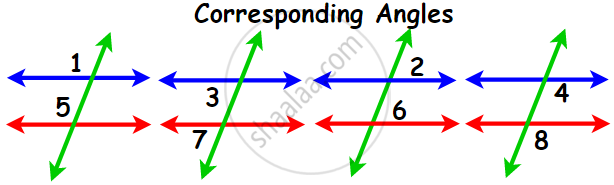## b. Alternate angles:

### (i) Alternate interior angles:

• Alternate interior angles have different vertices

• Alternate interior angles are on opposite sides of the transversal and

• Alternate interior angles lie ‘between’ the two lines i.e., both angles are interior.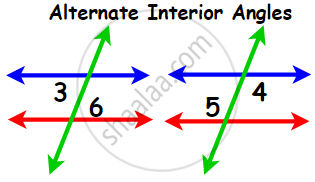### (ii) Alternate exterior angles:

• Alternate exterior angles have different vertices
• Alternate exterior angles are on opposite sides of the transversal
• Alternate exterior angles are not lain ‘between’ the two lines i.e., both angles are exterior.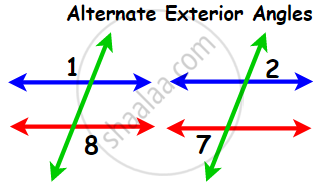The Z-shape stands for alternate angles: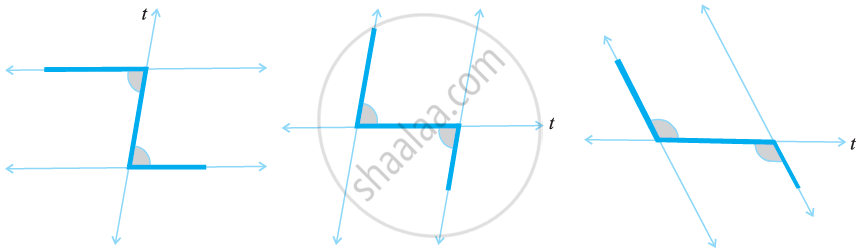## C. Consecutive Interior Angles:

• The pairs of angles on one side of the transversal but inside the two lines are called Consecutive Interior Angles.

• If the transversal cuts across parallel lines then the interior angles are supplementary (add to 180°) than you can conclude the lines are parallel.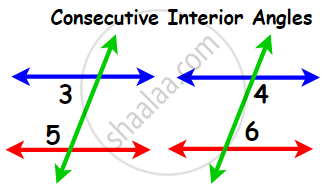# 2. Pairs of Lines - Angles Made by a Transversal:

• A transversal gives rise to several types of angles.
• Line l intersects lines m and n at points P and Q respectively. Therefore, line l is a transversal for lines m and n.
• ∠ 1, ∠ 2, ∠7, and ∠8 are called exterior angles, while ∠3, ∠4, ∠5, and ∠6 are called interior angles.
• Interior angles on the same side of the transversal are also referred to as consecutive interior angles or allied angles or co-interior angles.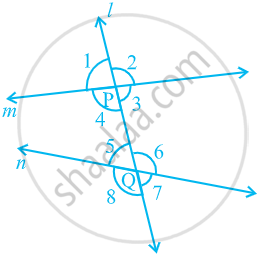(a) Corresponding angles:
(i) ∠ 1 and ∠ 5 (ii) ∠ 2 and ∠ 6
(iii) ∠ 4 and ∠ 8 (iv) ∠ 3 and ∠ 7

(b) Alternate interior angles:
(i) ∠ 4 and ∠ 6 (ii) ∠ 3 and ∠ 5

(c) Alternate exterior angles:
(i) ∠ 1 and ∠ 7 (ii) ∠ 2 and ∠ 8

(d) Interior angles on the same side of the transversal:
(i) ∠ 4 and ∠ 5 (ii) ∠ 3 and ∠ 6.

If you would like to contribute notes or other learning material, please submit them using the button below.

### Shaalaa.com

Angles Made by a Transversal [00:07:56]
S
0%